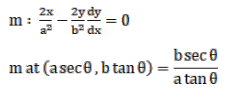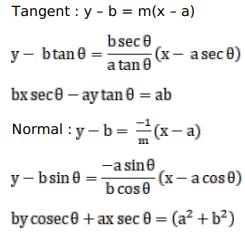# Find the equations of the tangent and the normal to the given curve at the indicated point for

Question:

Find the equations of the tangent and the normal to the given curve at the indicated point for

$\frac{x^{2}}{a^{2}}+\frac{y^{2}}{b^{2}}=1$ at $(a \sec \theta, b \tan \theta)$

Solution: